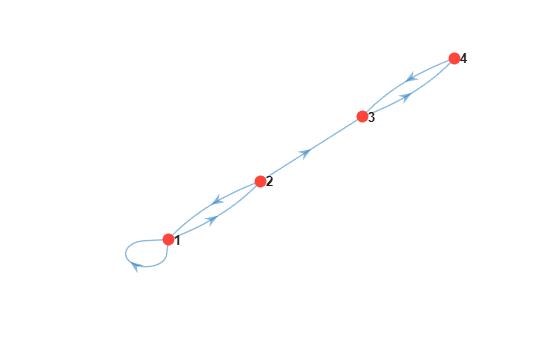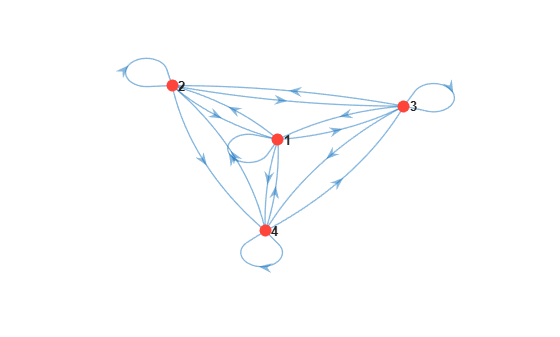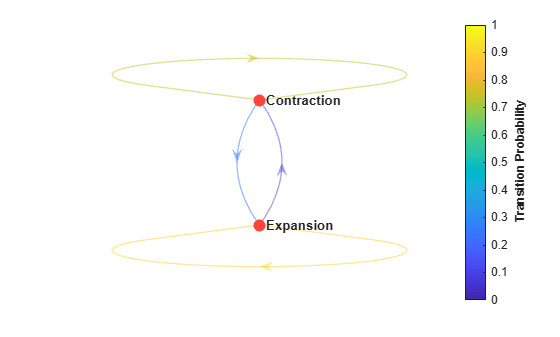# dtmc

Create discrete-time Markov chain

## Description

`dtmc` creates a discrete-time, finite-state, time-homogeneous Markov chain from a specified state transition matrix.

After creating a `dtmc` object, you can analyze the structure and evolution of the Markov chain, and visualize the Markov chain in various ways, by using the object functions.

## Creation

### Syntax

``mc = dtmc(P)``
``mc = dtmc(P,'StateNames',stateNames)``

### Description

example

``mc = dtmc(P)` creates the discrete-time Markov chain object `mc` specified by the state transition matrix `P`. `

example

``mc = dtmc(P,'StateNames',stateNames)` optionally associates the names `stateNames` to the states.`

### Input Arguments

expand all

State transition matrix, specified as a `numStates`-by-`numStates` nonnegative numeric matrix.

`P(i,j)` is either the theoretical probability of a transition from state `i` to state `j` or an empirical count of observed transitions from state `i` to state `j`. P can be fully specified (all elements are nonnegative numbers), partially specified (elements are a mix of nonnegative numbers and `NaN` values), or unknown (completely composed of `NaN` values).

`dtmc` normalizes each row of `P` without any `NaN` values to sum to `1`, then stores the normalized matrix in the property P.

Data Types: `double`

## Properties

expand all

You can set writable property values when you create the model object by using name-value pair argument syntax, or after you create the model object by using dot notation. For example, for the two-state model `mc`, to label the first and second states `Depression` and `Recession`, respectively, enter:

`mc.StateNames = ["Depression" "Recession"];`

Normalized transition matrix, specified as a `numStates`-by-`numStates` nonnegative numeric matrix.

If `x` is a row vector of length `numStates` specifying a distribution of states at time `t` (`x` sums to `1`), then `x*P` is the distribution of states at time `t + 1`.

`NaN` entries indicate estimable transition probabilities. The `estimate` function of `msVAR` treats the known elements of `P` as equality constraints during optimization.

Data Types: `double`

Number of states, specified as a positive scalar.

Data Types: `double`

State labels, specified as a string vector, cell vector of character vectors, or numeric vector of length `numStates`. Elements correspond to rows and columns of `P`.

Example: `["Depression" "Recession" "Stagnant" "Boom"]`

Data Types: `string`

## Object Functions

`dtmc` objects require a fully specified transition matrix `P`.

expand all

 `asymptotics` Determine Markov chain asymptotics `isergodic` Check Markov chain for ergodicity `isreducible` Check Markov chain for reducibility `classify` Classify Markov chain states `lazy` Adjust Markov chain state inertia `subchain` Extract Markov subchain
 `redistribute` Compute Markov chain redistributions `simulate` Simulate Markov chain state walks
 `distplot` Plot Markov chain redistributions `eigplot` Plot Markov chain eigenvalues `graphplot` Plot Markov chain directed graph `simplot` Plot Markov chain simulations

## Examples

collapse all

Consider this theoretical, right-stochastic transition matrix of a stochastic process.

`$P=\left[\begin{array}{cccc}0.5& 0.5& 0& 0\\ 0.5& 0& 0.5& 0\\ 0& 0& 0& 1\\ 0& 0& 1& 0\end{array}\right].$`

Element ${P}_{ij}$ is the probability that the process transitions to state j at time t + 1 given that it is in state i at time t, for all t.

Create the Markov chain that is characterized by the transition matrix P.

```P = [0.5 0.5 0 0; 0.5 0 0.5 0; 0 0 0 1; 0 0 1 0]; mc = dtmc(P);```

`mc` is a `dtmc` object that represents the Markov chain.

Display the number of states in the Markov chain.

`numstates = mc.NumStates`
```numstates = 4 ```

Plot a directed graph of the Markov chain.

```figure; graphplot(mc);```Observe that states 3 and 4 form an absorbing class, while states 1 and 2 are transient.

Consider this transition matrix in which element $\left(i,j\right)$ is the observed number of times state i transitions to state j.

`$P=\left[\begin{array}{cccc}16& 2& 3& 13\\ 5& 11& 10& 8\\ 9& 7& 6& 12\\ 4& 14& 15& 1\end{array}\right].$`

For example, ${P}_{32}=7$ implies that state 3 transitions to state 2 seven times.

```P = [16 2 3 13; 5 11 10 8; 9 7 6 12; 4 14 15 1];```

Create the Markov chain that is characterized by the transition matrix P.

`mc = dtmc(P);`

Display the normalized transition matrix stored in mc. Verify that the elements within rows sum to `1` for all rows.

`mc.P`
```ans = 4×4 0.4706 0.0588 0.0882 0.3824 0.1471 0.3235 0.2941 0.2353 0.2647 0.2059 0.1765 0.3529 0.1176 0.4118 0.4412 0.0294 ```
`sum(mc.P,2)`
```ans = 4×1 1 1 1 1 ```

Plot a directed graph of the Markov chain.

```figure; graphplot(mc);```Consider the two-state business cycle of the US real gross national product (GNP) in  p. 697. At time t, real GNP can be in a state of expansion or contraction. Suppose that the following statements are true during the sample period.

• If real GNP is expanding at time t, then the probability that it will continue in an expansion state at time t + 1 is ${p}_{11}=0.90$.

• If real GNP is contracting at time t, then the probability that it will continue in a contraction state at time t + 1 is ${p}_{22}=0.75$.

Create the transition matrix for the model.

```p11 = 0.90; p22 = 0.75; P = [p11 (1 - p11); (1 - p22) p22];```

Create the Markov chain that is characterized by the transition matrix P. Label the two states.

`mc = dtmc(P,'StateNames',["Expansion" "Contraction"])`
```mc = dtmc with properties: P: [2x2 double] StateNames: ["Expansion" "Contraction"] NumStates: 2 ```

Plot a directed graph of the Markov chain. Indicate the probability of transition by using edge colors.

```figure; graphplot(mc,'ColorEdges',true);```To help you explore the `dtmc` object functions, `mcmix` creates a Markov chain from a random transition matrix using only a specified number of states.

Create a five-state Markov chain from a random transition matrix.

```rng(1); % For reproducibility mc = mcmix(5)```
```mc = dtmc with properties: P: [5x5 double] StateNames: ["1" "2" "3" "4" "5"] NumStates: 5 ```

`mc` is a `dtmc` object.

Plot the eigenvalues of the transition matrix on the complex plane.

```figure; eigplot(mc)```This spectrum determines structural properties of the Markov chain, such as periodicity and mixing rate.

Consider a Markov-switching autoregression (`msVAR`) model for the US GDP containing four economic regimes: depression, recession, stagnation, and expansion. To estimate the transition probabilities of the switching mechanism, you must supply a `dtmc` model with an unknown transition matrix entries to the `msVAR` framework.

Create a 4-regime Markov chain with an unknown transition matrix (all `NaN` entries). Specify the regime names.

```P = nan(4); statenames = ["Depression" "Recession" ... "Stagnation" "Expansion"]; mcUnknown = dtmc(P,'StateNames',statenames)```
```mcUnknown = dtmc with properties: P: [4x4 double] StateNames: ["Depression" "Recession" "Stagnation" "Expansion"] NumStates: 4 ```
`mcUnknown.P`
```ans = 4×4 NaN NaN NaN NaN NaN NaN NaN NaN NaN NaN NaN NaN NaN NaN NaN NaN ```

Suppose economic theory states that the US economy never transitions to an expansion from a recession or depression. Create a 4-regime Markov chain with a partially known transition matrix representing the situation.

```P(1,4) = 0; P(2,4) = 0; mcPartial = dtmc(P,'StateNames',statenames)```
```mcPartial = dtmc with properties: P: [4x4 double] StateNames: ["Depression" "Recession" "Stagnation" "Expansion"] NumStates: 4 ```
`mcPartial.P`
```ans = 4×4 NaN NaN NaN 0 NaN NaN NaN 0 NaN NaN NaN NaN NaN NaN NaN NaN ```

The `estimate` function of `msVAR` treats the known elements of `mcPartial.P` as equality constraints during optimization.

For more details on Markov-switching dynamic regression models, see `msVAR`.

## Alternatives

You also can create a Markov chain object using `mcmix`.

 Gallager, R.G. Stochastic Processes: Theory for Applications. Cambridge, UK: Cambridge University Press, 2013.

 Haggstrom, O. Finite Markov Chains and Algorithmic Applications. Cambridge, UK: Cambridge University Press, 2002.

 Hamilton, J. D. Time Series Analysis. Princeton, NJ: Princeton University Press, 1994.

 Norris, J. R. Markov Chains. Cambridge, UK: Cambridge University Press, 1997.